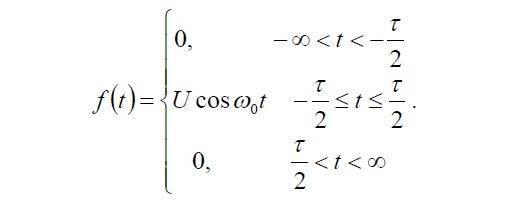# Finding the fourier spectrum of a function

• diredragon
In summary, the conversation is about finding the Fourier spectrum of a function that is the product of a rectangular impulse and a cosine function. The solution provided uses the complex Fourier coefficient formula and the property of the Fourier series. To draw the graph, one can plot two sinc-functions centered around the positions of the delta-functions.

## Homework Statement

Find the Fourier spectrum ##C_k## of the following function and draw it's graph:## Homework Equations

3. The Attempt at a Solution [/B]
I know that the complex Fourier coefficient of a rectangular impulse ##U## on an interval ##[-\frac{\tau}{2}, \frac{\tau}{2}]## is ##C_k = \frac{U\tau}{T}\frac{\sin {kw\frac{\tau}{2}}}{kw\frac{\tau}{2}}## and since ##f(t)=U\cos {w_ot}## i can say that ##f(t)=\frac{U}{2}(e^{jw_ot}-e^{-jw_ot})## which if i use the property of the Fourier series get:
##C_k = \frac{U\tau}{T}\frac{\sin {k(w+w_o)\frac{\tau}{2}}}{k(w+w_o)\frac{\tau}{2}} - \frac{U\tau}{T}\frac{\sin {k(w-w_o)\frac{\tau}{2}}}{k(w-w_o)\frac{\tau}{2}}##. Is this correct. How would i draw a graph of this?

#### Attachments

I think you have the solution. You can also think of it as follows: Your function is the product of a rectangle and a cosine. The FT of the rectangle is the sinc-function that you have in your solution. The FT of the cosine consists of two delta-functions (at plus and minus the frequency of the cosine). The FT of the product is the convolution of the two separate FTs and that's what you write. The graph should show two sinc-functions centered around the positions of the deltas.

•Merlin3189 and diredragon